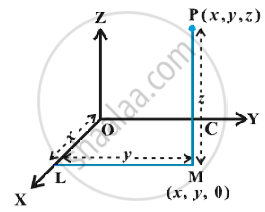# Three - Dimensional Geometry - Coordinates of a Point in Space

#### notes

Now explain a point in the space , with three coordinates (x,y,z) and conversely, given a triplet of three numbers (x, y, z).
Given a point P in space , we drop a perpendicular PM on the XY-plane with M as the foot of this perpendicular Fig.Then, from the point M, we draw a perpendicular ML to the x-axis, meeting it at L. Let OL be x, LM be y and MP be z. Then x,y and z are called the x, y and z coordinates, respectively, of the point P in the space.  the point P (x, y, z) lies in the octant XOYZ and so all x, y, z are positive.  If P was in any other octant, the signs of x, y and z would change accordingly. Thus, to each point P in the space there corresponds an ordered triplet (x, y, z) of real numbers.
Conversely , given any triplet (x,y,z) , we would first the point L on the x-axis corresponding to x, then locate the point M in the XY-plane such that (x, y) are the coordinates of the point M in the XY-plane.  Note that LM is perpendicular to the x-axis or is parallel to the y-axis. Having reached the point M, we draw a perpendicular MP to the XY-plane and locate on it the point P corresponding to z. The point P so obtained has then the coordinates (x, y, z). Thus, there is a one to one correspondence between the points in space and ordered triplet (x, y, z) of real numbers.

If you would like to contribute notes or other learning material, please submit them using the button below.

### Shaalaa.com

Coordinates of a Point in Space [00:00:00]
S
0%